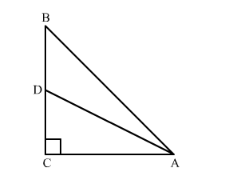# In right-angled triangle ABC is which ∠C = 90°,

Question:

In right-angled triangle $\mathrm{ABC}$ is which $\angle \mathrm{C}=90^{\circ}$, if $\mathrm{D}$ is the mid-point of $\mathrm{BC}$, prove that $\mathrm{AB}^{2}=4 \mathrm{AD}^{2}-3 \mathrm{AC}^{2}$.

Solution:

$\triangle \mathrm{ABC}$ is a right-angled triangle with $\angle \mathrm{C}=90^{\circ}$. D is the mid-point of $\mathrm{BC}$.

We need to prove that $A B^{2}=4 A D^{2}-3 A C^{2}$.Since D is the midpoint of the side BC, we get

BD = DC

$\therefore B C=2 D C$

Using Pythagoras theorem in triangles right angled triangle ABC

$A B^{2}=A C^{2}+B C^{2}$

$A B^{2}=A C^{2}+(2 D C)^{2}$

$A B^{2}=A C^{2}+4 D C^{2}$

Again using Pythagoras theorem in the right angled triangle ADC

$A D^{2}=A C^{2}+D C^{2}$

$D C^{2}=A D^{2}-A C^{2}$....(2)

From (1) and (2), we get

$A B^{2}=A C^{2}+4\left(A D^{2}-A C^{2}\right)$

$A B^{2}=A C^{2}+4 A D^{2}-4 A C^{2}$

$A B^{2}=4 A D^{2}-3 A C^{2}$

Hence, $A B^{2}=4 A D^{2}-3 A C^{2}$.